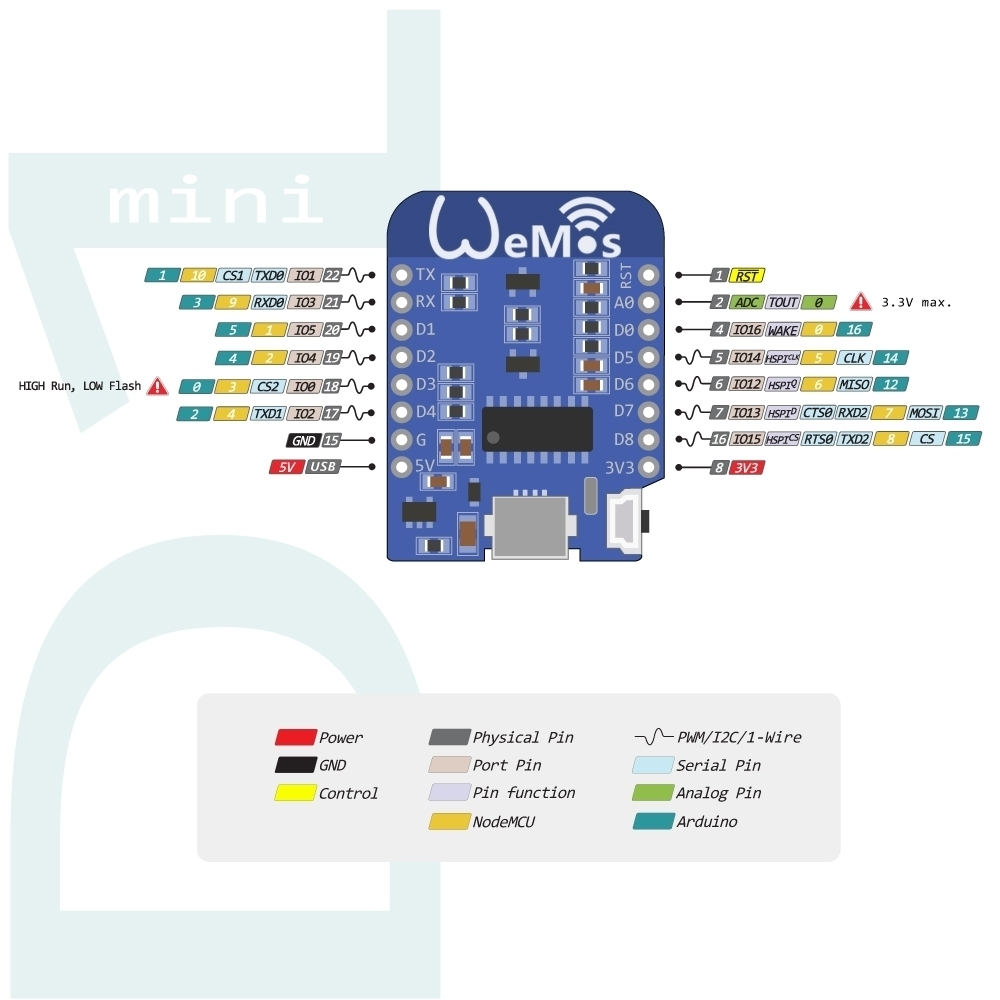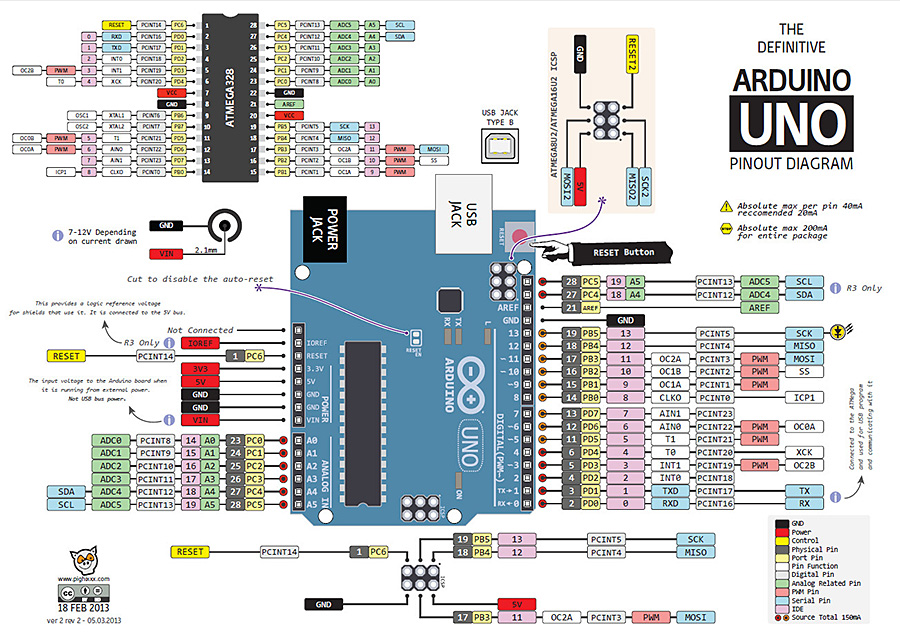# B4R TutorialArduino to WeMos pin mapping

Hello,
Not exactly a tutorial, but informative nevertheless.

This is how to map your Arduino projects to your WeMos. So for example in the blink demo, you just choose pin 2 for a WeMos instead of pin 13 for the Arduino.

Below are the mapped pins:
Arduino = 0 -> WeMos = D3
Arduino = 1 -> WeMos= NC
Arduino = 2 -> WeMos= D4 (LED)
Arduino = 3 -> WeMos= NC
Arduino = 4 -> WeMos= D2
Arduino = 5 -> WeMos= D1
Arduino = 6 -> WeMos= Watchdog(4) Reset
Arduino = 7 -> WeMos= Watchdog(4) Reset
Arduino = 8 -> WeMos= Watchdog(4) Reset
Arduino = 9 -> WeMos= Watchdog(4) Reset
Arduino = 10 -> WeMos= NC
Arduino = 11 -> WeMos= Watchdog(4) Reset
Arduino = 12 -> WeMos= D7 low, D6 sporadic
Arduino = 13 -> WeMos= D0 high, D7 sporadic
Arduino = 14 -> WeMos= D0 high, D6 Low, D7 Low, D5 Sporadic
Arduino = 15 -> WeMos= High, D8 Sporadic
Arduino = 16 -> WeMos= D0
Arduino = 17 -> WeMos= NC
Arduino = 18 -> WeMos= NC
Arduino = 19 -> WeMos= NC
Arduino = 20 -> WeMos= Stack Trace and Pin-Based(2) Reset
Arduino = 21 -> WeMos= Stack Trace and Watchdog(4) Reset
Arduino = 22 -> WeMos= Stack Trace and Watchdog(4) Reset
Arduino = 23 -> WeMos= Stack Trace and Watchdog(4) Reset
Arduino = 24 -> WeMos= NC
Arduino = 25 -> WeMos= NC
Arduino = 26 -> WeMos= NC
Arduino = 27 -> WeMos= NC
Arduino = 28 -> WeMos= NC
Arduino = 29 -> WeMos= NC

A visual representation WeMos D1 Mini:A visual representation Arduino Uno R3:Last edited: